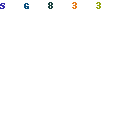Sine/Cosine wave
oscillatorI introduce the oscillator which outputs the Sine wave and the Cosine wave at the same time using the operational amplifier.
Because the distortion was big at the circuit to have been introducing before, I improved at the circuit with little distortion.
The circuit this time combines the integration circuit and the inverter by the operational amplifier.
The 90 degree phase is shifted about the sine wave and the cosine wave. The sine wave is the signal to be 90 degree delayed from the cosine wave.

In case of C=C1=C2, R=R4=R5, the oscillation frequency can be calculated by the following formula.The example of the circuit which was made this time is shown below.
 f = 1/(2 x 3.14 x 0.001 x 10-6 x 15 x 103) = 1/(0.0942 x 10-3) = 10.62 x 103 = 10.62 KHz

The frequency in the actual circuit was to 10.585 KHz be.Pattern drawingOperation explanation